The requested page is not available for the requested platform. You are viewing the content for Default platform.

19.2
19.1
18.2
18.1
17.2

# Window Calculation Functions

This topic contains a description of window functions that can be used to specify measure expressions.

Function

Description

Example

Last()

Returns the number of rows from the current row to the last row in the window.

Last()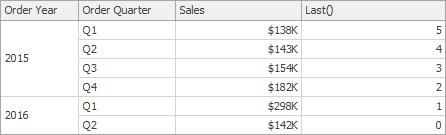First()

Returns the number of rows from the current row to the first row in the window.

First()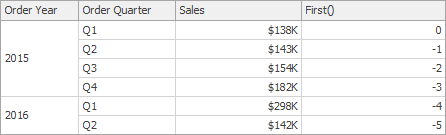Index()

Returns the index of the current row in the window.

Index()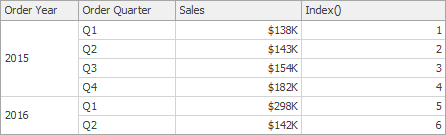Size()

Returns the number of rows in the window.

Size()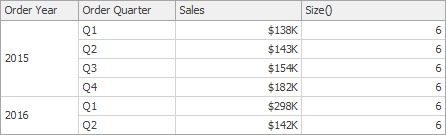Lookup(SummaryExpression, Position)

Returns the value of the expression in a target position specified as a relative offset from the current position.

Lookup(Sum([Sales]), 3)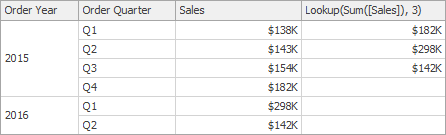RankCompetition(SummaryExpression, [ 'asc' | 'desc' ])

Returns the standard competition rank for the current row in the window.

RankCompetition(Sum([Sales]), 'asc')

RankDense(SummaryExpression, [ 'asc' | 'desc' ])

Returns the dense rank for the current row in the window.

RankDense(Sum([Sales]), 'asc')

RankUnique(SummaryExpression, [ 'asc' | 'desc' ])

Returns the unique rank for the current row in the window.

RankUnique(Sum([Sales]), 'asc')

RankModified(SummaryExpression, [ 'asc' | 'desc' ])

Returns the modified competition rank for the current row in the window.

RankModified(Sum([Sales]), 'asc')

RankPercentile(SummaryExpression, [ 'asc' | 'desc' ])

Returns the percentile rank for the current row in the window.

RankPercentile(Sum([Sales]), 'desc')

RunningAvg(SummaryExpression)

Returns the running average of the specified expression from the first row in the window to the current row.

RunningAvg(Sum([Sales]))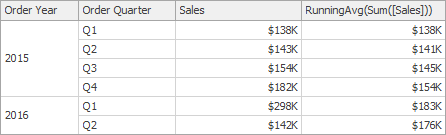RunningCount(SummaryExpression)

Returns the running count of the specified expression from the first row in the window to the current row.

RunningCount(Sum([Sales]))

RunningMax(SummaryExpression)

Returns the running maximum of the specified expression from the first row in the window to the current row.

RunningMax(Sum([Sales]))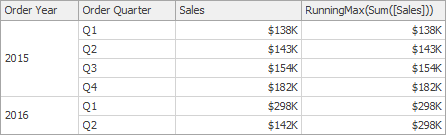RunningMin(SummaryExpression)

Returns the running minimum of the specified expression from the first row in the window to the current row.

RunningMin(Sum([Sales]))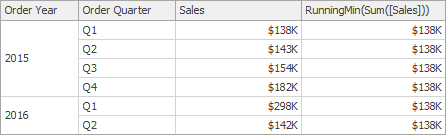RunningSum(SummaryExpression)

Returns the running sum of the specified expression from the first row in the window to the current row.

RunningSum(Sum([Sales]))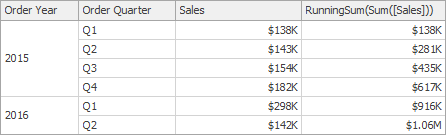WindowAvg(SummaryExpression, StartOffset, EndOffset)

Returns the average of the expression within the window, which is defined using offsets from the current row.

WindowAvg(Sum([Sales]), First(), Last())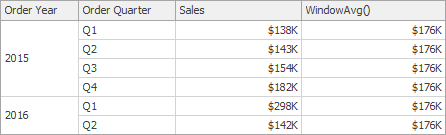WindowCount(SummaryExpression, StartOffset, EndOffset)

Returns the count of the expression within the window.

WindowCount(Sum([Sales]), First()+2, Last())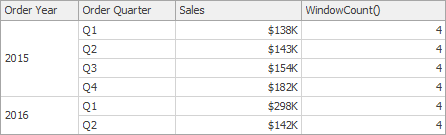WindowCountDistinct(SummaryExpression, StartOffset, EndOffset)

Returns the distinct count of the expression within the window.

WindowCountDistinct(Sum([Sales]), First(), Last())

WindowMax(SummaryExpression, StartOffset, EndOffset)

Returns the maximum of the expression within the window.

WindowMax(Sum([Sales]), First(), Last())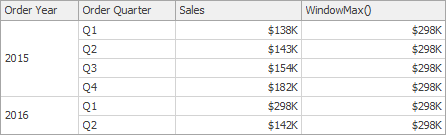WindowMin(SummaryExpression, StartOffset, EndOffset)

Returns the minimum of the expression within the window.

WindowMin(Sum([Sales]), First(), Last())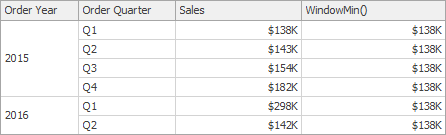WindowMode(SummaryExpression, StartOffset, EndOffset)

Returns the statistical mode in the window (the element that occurs most often in the values calculated with the specified expression within the window).

WindowMode(Sum([Sales]), First(), Last())

WindowMedian(SummaryExpression, StartOffset, EndOffset)

Returns the median of the expression within the window.

WindowMedian(Sum([Sales]), First(), Last())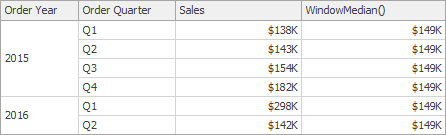WindowSum(SummaryExpression, StartOffset, EndOffset)

Returns the sum of the expression within the window.

WindowSum(Sum([Sales]), First()+2, Last())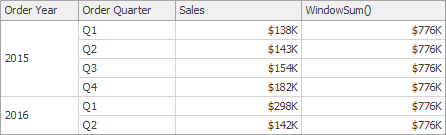WindowVar(SummaryExpression, StartOffset, EndOffset)

Returns the variance of the expression within the window.

WindowVar(Sum([Sales]), First(), Last())

WindowVarp(SummaryExpression, StartOffset, EndOffset)

Returns the biased variance of the expression within the window.

WindowVarp(Sum([Sales]), First(), Last())

WindowStdDev(SummaryExpression, StartOffset, EndOffset)

Returns the sample standard deviation of the expression within the window.

WindowStdDev(Sum([Sales]), First(), Last())

WindowStdDevp(SummaryExpression, StartOffset, EndOffset)

Returns the biased standard deviation of the expression within the window.

WindowStdDevp(Sum([Sales]), First(), Last())

Total(SummaryExpression)

Returns the total for the specified expression in a calculation window.

##### Note

Note that the Total function calculates the total based on values from the underlying data source.

Total(Sum([Sales]))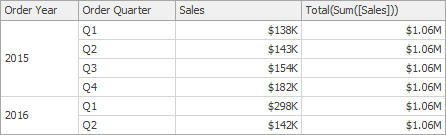##### Important

Note that window functions cannot be used inside Aggr.